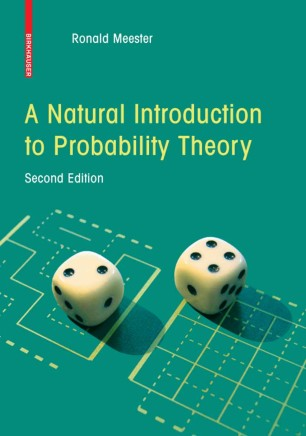# A Natural Introduction to Probability Theory

## Benefits

• Discovering of the theory along the way, rather than presenting it matter of factly at the beginning

• Contains many original and surprising examples

• A rigorous study without any measure theory

• Compactly written, but nevertheless very readable

• A probabilistic approach, appealing to intuition, introducing technical machinery only when necessaryTextbook

1. Front Matter
Pages i-x
2. Pages 1-33
3. Pages 35-70
4. Pages 71-79
5. Pages 81-88
6. Pages 89-92
7. Pages 93-135
8. Pages 137-151
9. Pages 153-166
10. Pages 167-182
11. Pages 183-186
12. Back Matter
Pages 187-197

### Introduction

According to Leo Breiman (1968), probability theory has a right and a left hand. The right hand refers to rigorous mathematics, and the left hand refers to ‘pro- bilistic thinking’. The combination of these two aspects makes probability theory one of the most exciting ?elds in mathematics. One can study probability as a purely mathematical enterprise, but even when you do that, all the concepts that arisedo haveameaningontheintuitivelevel.Forinstance,wehaveto de?newhat we mean exactly by independent events as a mathematical concept, but clearly, we all know that when we ?ip a coin twice, the event that the ?rst gives heads is independent of the event that the second gives tails. Why have I written this book? I have been teaching probability for more than ?fteen years now, and decided to do something with this experience. There are already many introductory texts about probability, and there had better be a good reason to write a new one. I will try to explain my reasons now.

### Keywords

Excel Limit theorems Probability theory Random variable Random walk coding mathematical statistics measure theory

#### Authors and affiliations

1. 1.Faculteit der Exacte WetenschappenVrije UniversiteitAmsterdamThe Netherlands

### Bibliographic information

• Book Title A Natural Introduction to Probability Theory
• Authors R. Meester
• DOI https://doi.org/10.1007/978-3-7643-8724-2
• Copyright Information Birkhäuser Verlag AG 2008
• Publisher Name Birkhäuser Basel
• eBook Packages Mathematics and Statistics Mathematics and Statistics (R0)
• Softcover ISBN 978-3-7643-8723-5
• eBook ISBN 978-3-7643-8724-2
• Edition Number 2
• Number of Pages X, 198
• Number of Illustrations 0 b/w illustrations, 0 illustrations in colour
• Topics
• Buy this book on publisher's site
Industry Sectors
Pharma
Biotechnology
IT & Software
Telecommunications
Electronics
Energy, Utilities & Environment
Aerospace
Oil, Gas & Geosciences
Engineering

## Reviews

"The book [is] an excellent new introductory text on probability. The classical way of teaching probability is based on measure theory. In this book discrete and continuous probability are studied with mathematical precision, within the realm of Riemann integration and not using notions from measure theory…. Numerous topics are discussed, such as: random walks, weak laws of large numbers, infinitely many repetitions, strong laws of large numbers, branching processes, weak convergence and [the] central limit theorem. The theory is illustrated with many original and surprising examples and problems."

—ZENTRALBLATT MATH

"Most textbooks designed for a one-year course in mathematical statistics cover probability in the first few chapters as preparation for the statistics to come. This book in some ways resembles the first part of such textbooks: it's all probability, no statistics. But it does the probability more fully than usual, spending lots of time on motivation, explanation, and rigorous development of the mathematics…. The exposition is usually clear and eloquent…. Overall, this is a five-star book on probability that could be used as a textbook or as a supplement."

—MAA ONLINE

"It seems that a task to provide an introductory course on probablitity fulfilling the following requirements arises not so rarely: (A) The course should be accessible to studnets having only very modest preliminary knowledge of calculus, in particular, with no acquaintance with measure theory. (B) The presentation should be fully rigorous. (C) Nontrivial resuilts should be give. (D) Motivation for further strudy of measure theoretic probability ought to be provided, hence to contetn oneself to countable probability spaces is undesirable.  R. Meester's book is an attametp to shot that all these demands may be fulfilled in a reasonalb eway, however incompatible they may look at first sight."

---Mathematica Bohemica